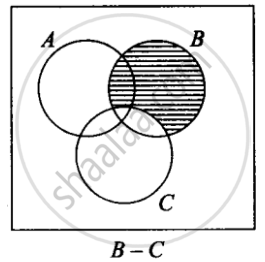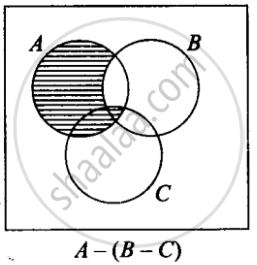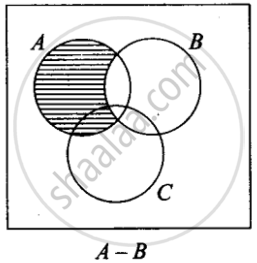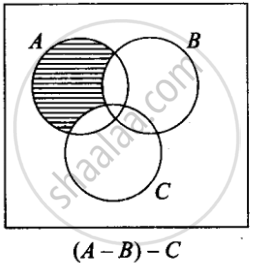# Determine whether the following statement is true or false. Justify your answer. For all sets A, B, and C, A – (B – C) = (A – B) – C - Mathematics

MCQ
True or False

Determine whether the following statement is true or false. Justify your answer.

For all sets A, B, and C, A – (B – C) = (A – B) – C

• True

• False

#### Solution

This statement is False.

Explanation:From the Venn Diagrams

A – (B – C) ≠ (A – B) – C

Concept: Sets and Their Representations
Is there an error in this question or solution?

#### APPEARS IN

NCERT Mathematics Exemplar Class 11
Chapter 1 Sets
Exercise | Q 14 | Page 14

Share